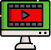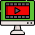VideosNotes

### Approximation

Anti-Logarithm
The anti-logarithm of a number is the inverse process of finding the logarithms of the same number. If x is the logarithm of a number y with a given base b, then y is the anti-logarithm of (antilog) of x to the base b. Natural Logarithms and Anti-Logarithms have their base as 2.7183
Views: N/A
Video Duration: 9:33
Date Uploaded: November 03, 2020
Grade Level: SS 2
Share this with your friends!
On the Same TopicLOGARITHMS
Logarithm, the exponent or ...Logarithmic Equations
i. Revision of logarithm of...Logarithm - Quotient Rule
Since eln(x/y)=eln(x)−ln(y)...LOGARITHMS NUMBERS
Logarithm, the exponent or ...
Other Related Videos